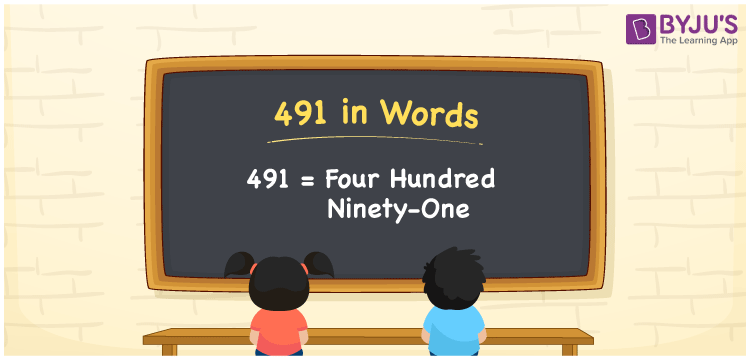# 491 in Words

491 in words can be written as Four Hundred Ninety-one. The concepts like counting numbers which are important from primary education are discussed here in brief. For instance, if you spend Rs. 491 in buying top, then you can say that “I bought a top for Four Hundred Ninety-one Rupees”. The English alphabet is used to write 491 in words. Students can get a clear knowledge of numbers in words with the help of the study materials given at BYJU’S. The number 491 can be read as “Four Hundred Ninety-one” in English.

 491 in words Four Hundred Ninety-one Four Hundred Ninety-one in Numbers 491

## 491 in English Words## How to Write 491 in Words?

Place value chart and expanded form of 491 can be learnt easily in this section. There are three digits in the number 491. The place value chart for 491 is provided below for reference to help students understand the concepts effectively.

 Hundreds Tens Ones 4 9 1

The expanded form of 491 is provided below:

4 × Hundred + 9 × Ten + 1 × One

= 4 × 100 + 9 × 10 + 1 × 1

= 491

= Four Hundred Ninety-one

Hence, 491 in words is written as Four Hundred Ninety-one.

491 is a natural number that precedes 492 and succeeds 490.

491 in words – Four Hundred Ninety-one

Is 491 an odd number? – Yes

Is 491 an even number? – No

Is 491 a perfect square number? – No

Is 491 a perfect cube number? – No

Is 491 a prime number? – Yes

Is 491 a composite number? – No

## Frequently Asked Questions on 491 in Words

Q1

### How can 491 be written in words?

491 can be written as “Four Hundred Ninety-one” in words.
Q2

### Is 491 an odd number?

No, 491 is an odd number because it is not divisible by 2.
Q3

### Write Four Hundred Ninety-one in numbers.

Four Hundred Ninety-one can be written in numbers as 491.